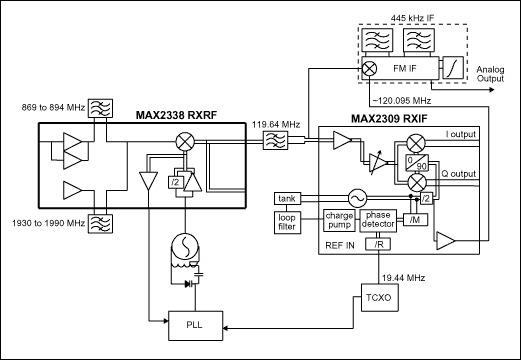# 优化双模蜂窝电话中MAX2309 PLL分频比

### 引言### 用于数字模式的第二本振

1. 将VCO远离从第一中频。
2. 更精确地获得50%的占空比。
3. 简化正交解调器中的90度移相电路。

• R = 486, M = 5982 fREF (相位检测器的工作级) = 19.44MHz/486 = 40kHz
• R = 243, M = 2991 fREF = 80kHz

### 用于AMPS的第二本振

1. 记下所需的本振，本例中为120.095MHz。
2. 调整本振频率值以解决2分频的功能实现， VCO = 240.19MHz。
3. 注意VCO频率中出现最后整数的位置，确定VCO出现最后整数位置是在10kHz上；这个值作为鉴相器比较频率的候选值—fREF = 10kHz。还有其它办法来解决问题，但是通常情况下这些其它速率很难直接观察出来。
4. 确定时基，TCXO应为fREF的整数倍，19.44MHz / 10kHz = 1944。
5. 计算R值，参考分频器；1944这个值由第4步得到。
6. 计算M值，反馈分频器；240.19MHz / 10kHz = 24,019。

### 结果

R = 422, M = 5214，输出频率为：19.44MHz × (5214/422) = 240.189952607 ，误差约47Hz

### 附录

fTCXO:= 19.44 × 106；PLL参考频率
fTARGET:= 240.19 × 106； 所期望的或目标输出频率(在第二混频器下的高端注入)。

R的最大值将把PLL相位检测器参考值设为10kHz，这样通过简单整数M将输出频率设置为期望值。考虑的R的最小值为使鉴相器运行在最大允许频率下的一个值。对于这种情况，假设检测器的最大运行速率为约1MHz。

StepSize:= 10 × 10³

RMIN:= fTCXO / 1 × 106

RMIN = 19.44；因为实际的硬件逻辑限制，所以此值必须为整数。转换为整数。

RMIN:= RMIN - mod(RMIN, 1) ；将实数转换为整数。

RMIN = 19

RMAX:= fTCXO / StepSize ；估算参考分频器最大的分频值

RMAX:= RMAX - mod(RMAX, 1)

RMAX = 1944

MMIN:= fTARGET × RMIN / fTCXO

MMIN:= MMIN - mod(MMIN, 1)

MMIN = 234

MMAX:= fTARGET× RMAX / fTCXO

MMAX:= MMAX - mod(MMAX, 1)

MMAX = 24019

MaxAttempts:= (RMAX - RMIN) × (MMAX - MMIN)

MaxAttempts = 4.579 × 107

ε := 50；允许的频率误差

R:= RMIN, RMIN +1...RMAX ；设置R值的搜索范围

Me (R) = fTARGET × R / fTCXO

intMe (R):= Me (R) - mod(Me(R), 1)

fOUT(R):= intMe(R) × fTCXO / R

g(R):= if [ (fout(R) - ftarget)² < ε ², R, 0]鉴相器比较 频率 g(211) = 211 fTCXO / 211 = 92132.701 g(422) = 422 fTCXO / 422 = 46066.351 g(633) = 633 fTCXO / 633 = 30710.9 g(844) = 844 fTCXO / 844 = 23033.175 g(1055) = 1055 fTCXO / 1055 = 18426.54 g(126) = 1266 fTCXO / 1266 = 15355.45

### 参考资料

1. Coffman, Ken Real World FPGA Design with Verilog, Prentice Hall PTR, Upper Saddle River, NJ, 2000, pg. 71.